The LOGISTIC Procedure
 Input and Output Data Sets

OUTEST= Output Data Set

The OUTEST= data set contains one observation for each BY group containing the maximum likelihood estimates of the regression coefficients. If you also use the COVOUT option in the PROC LOGISTIC statement, there are additional observations containing the rows of the estimated covariance matrix. If you specify SELECTION=FORWARD, BACKWARD, or STEPWISE, only the estimates of the parameters and covariance matrix for the final model are output to the OUTEST= data set.

Variables in the OUTEST= Data Set

The OUTEST= data set contains the following variables:

• any BY variables specified

• _LINK_, a character variable of length 8 with four possible values: CLOGLOG for the complementary log-log function, LOGIT for the logit function, NORMIT for the probit (alias normit) function, and GLOGIT for the generalized logit function

• _TYPE_, a character variable of length 8 with two possible values: PARMS for parameter estimates or COV for covariance estimates. If an EXACT statement is also specified, then two other values are possible: EPARMMLE for the exact maximum likelihood estimates and EPARMMUE for the exact median unbiased estimates.

• _NAME_, a character variable containing the name of the response variable when _TYPE_=PARMS, EPARMMLE, and EPARMMUE, or the name of a model parameter when _TYPE_=COV

• _STATUS_, a character variable that indicates whether the estimates have converged

• one variable for each intercept parameter

• one variable for each slope parameter and one variable for the offset variable if the OFFSET= option if specified. If an effect is not included in the final model in a model building process, the corresponding parameter estimates and covariances are set to missing values.

• _LNLIKE_, the log likelihood

Parameter Names in the OUTEST= Data Set

If there are only two response categories in the entire data set, the intercept parameter is named Intercept. If there are more than two response categories in the entire data set, the intercept parameters are named Intercept_xxx, where xxx is the value (formatted if a format is applied) of the corresponding response category.

For continuous explanatory variables, the names of the parameters are the same as the corresponding variables. For CLASS variables, the parameter names are obtained by concatenating the corresponding CLASS variable name with the CLASS category; see the section Class Variable Naming Convention for more details. For interaction and nested effects, the parameter names are created by concatenating the names of each effect.

For the generalized logit model, names of parameters corresponding to each nonreference category contain _xxx as the suffix, where xxx is the value (formatted if a format is applied) of the corresponding nonreference category. For example, suppose the variable Net3 represents the television network (ABC, CBS, and NBC) viewed at a certain time. The following statements fit a generalized logit model with Age and Gender (a CLASS variable with values Female and Male) as explanatory variables:

proc logistic;
class Gender;
model Net3 = Age Gender / link=glogit;
run;

There are two logit functions, one contrasting ABC with NBC and the other contrasting CBS with NBC. For each logit, there are three parameters: an intercept parameter, a slope parameter for Age, and a slope parameter for Gender (since there are only two gender levels and the EFFECT parameterization is used by default). The names of the parameters and their descriptions are as follows:

Intercept_ABC

intercept parameter for the logit contrasting ABC with NBC

Intercept_CBS

intercept parameter for the logit contrasting CBS with NBC

Age_ABC

Age slope parameter for the logit contrasting ABC with NBC

Age_CBS

Age slope parameter for the logit contrasting CBS with NBC

GenderFemale_ABC

Gender=Female slope parameter for the logit contrasting ABC with NBC

GenderFemale_CBS

Gender=Female slope parameter for the logit contrasting CBS with NBC

INEST= Input Data Set

You can specify starting values for the iterative algorithm in the INEST= data set. The INEST= data set has the same structure as the OUTEST= data set but is not required to have all the variables or observations that appear in the OUTEST= data set. A previous OUTEST= data set can be used as, or modified for use as, an INEST= data set.

The INEST= data set must contain the intercept variables (named Intercept for binary response models and Intercept, Intercept_2, Intercept_3, and so forth, for ordinal and nominal response models) and all explanatory variables in the MODEL statement. If BY processing is used, the INEST= data set should also include the BY variables, and there must be one observation for each BY group. If the INEST= data set also contains the _TYPE_ variable, only observations with _TYPE_ value ’PARMS’ are used as starting values.

OUT= Output Data Set in the OUTPUT Statement

The OUT= data set in the OUTPUT statement contains all the variables in the input data set along with statistics you request by specifying keyword=name options or the PREDPROBS= option in the OUTPUT statement. In addition, if you use the single-trial syntax and you request any of the XBETA=, STDXBETA=, PREDICTED=, LCL=, and UCL= options, the OUT= data set contains the automatic variable _LEVEL_. The value of _LEVEL_ identifies the response category upon which the computed values of XBETA=, STDXBETA=, PREDICTED=, LCL=, and UCL= are based.

When there are more than two response levels, only variables named by the XBETA=, STDXBETA=, PREDICTED=, LOWER=, and UPPER= options and the variables given by PREDPROBS=(INDIVIDUAL CUMULATIVE) have their values computed; the other variables have missing values. If you fit a generalized logit model, the cumulative predicted probabilities are not computed.

When there are only two response categories, each input observation produces one observation in the OUT= data set.

If there are more than two response categories and you specify only the PREDPROBS= option, then each input observation produces one observation in the OUT= data set. However, if you fit an ordinal (cumulative) model and specify options other than the PREDPROBS= options, each input observation generates as many output observations as one fewer than the number of response levels, and the predicted probabilities and their confidence limits correspond to the cumulative predicted probabilities. If you fit a generalized logit model and specify options other than the PREDPROBS= options, each input observation generates as many output observations as the number of response categories; the predicted probabilities and their confidence limits correspond to the probabilities of individual response categories.

For observations in which only the response variable is missing, values of the XBETA=, STDXBETA=, PREDICTED=, UPPER=, LOWER=, and the PREDPROBS= options are computed even though these observations do not affect the model fit. This enables, for instance, predicted probabilities to be computed for new observations.

OUT= Output Data Set in a SCORE Statement

The OUT= data set in a SCORE statement contains all the variables in the data set being scored. The data set being scored can be either the input DATA= data set in the PROC LOGISTIC statement or the DATA= data set in the SCORE statement. The DATA= data set in the SCORE statement does not need to contain the response variable.

If the data set being scored contains the response variable, then denote the normalized levels (left-justified, formatted values of 16 characters or less) of your response variable Y by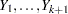. For each response level, the OUT= data set also contains the following:

• F_Y, the normalized levels of the response variable Y in the data set being scored. If the events/trials syntax is used, the F_Y variable is not created.

• I_Y, the normalized levels that the observations are classified into. Note that an observation is classified into the level with the largest probability. If the events/trials syntax is used, the _INTO_ variable is created instead, and it contains the values EVENT and NONEVENT.

• P_Y, the posterior probabilities of the normalized response level Y• If the CLM option is specified in the SCORE statement, the OUT= data set also includes the following:

• LCL_Y, the lower 100(
)% confidence limits for P_Y• UCL_Y, the upper 100()% confidence limits for P_YOUTDIST= Output Data Set

The OUTDIST= data set contains every exact conditional distribution necessary to process the corresponding EXACT statement. For example, the following statements create one distribution for the x1 parameter and another for the x2 parameters, and produce the data set dist shown in Table 51.10:

data test;
input y x1 x2 count;
datalines;
0 0 0 1
1 0 0 1
0 1 1 2
1 1 1 1
1 0 2 3
1 1 2 1
1 2 0 3
1 2 1 2
1 2 2 1
;
proc logistic data=test exactonly;
class x2 / param=ref;
model y=x1 x2;
exact x1 x2/ outdist=dist;
proc print data=dist;
run;
Table 51.10 OUTDIST= Data Set

Obs

x1

x20

x21

Count

Score

Prob

1

.

0

0

3

5.81151

0.03333

2

.

0

1

15

1.66031

0.16667

3

.

0

2

9

3.12728

0.10000

4

.

1

0

15

1.46523

0.16667

5

.

1

1

18

0.21675

0.20000

6

.

1

2

6

4.58644

0.06667

7

.

2

0

19

1.61869

0.21111

8

.

2

1

2

3.27293

0.02222

9

.

3

0

3

6.27189

0.03333

10

2

.

.

6

3.03030

0.12000

11

3

.

.

12

0.75758

0.24000

12

4

.

.

11

0.00000

0.22000

13

5

.

.

18

0.75758

0.36000

14

6

.

.

3

3.03030

0.06000

The first nine observations in the dist data set contain an exact distribution for the parameters of the x2 effect (hence the values for the x1 parameter are missing), and the remaining five observations are for the x1 parameter. If a joint distribution was created, there would be observations with values for both the x1 and x2 parameters. For CLASS variables, the corresponding parameters in the dist data set are identified by concatenating the variable name with the appropriate classification level.

The data set contains the possible sufficient statistics of the parameters for the effects specified in the EXACT statement, and the Count variable contains the number of different responses that yield these statistics. In particular, there are six possible response vectors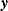for which the dot product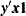was equal to 2, and for which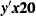,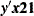, and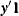were equal to their actual observed values (displayed in the "Sufficient Statistics" table).

When hypothesis tests are performed on the parameters, the Prob variable contains the probability of obtaining that statistic (which is just the count divided by the total count), and the Score variable contains the score for that statistic.

The OUTDIST= data set can contain a different exact conditional distribution for each specified EXACT statement. For example, consider the following EXACT statements:

exact 'O1'   x1    /           outdist=o1;
exact 'OJ12' x1 x2 / jointonly outdist=oj12;
exact 'OA12' x1 x2 / joint     outdist=oa12;
exact 'OE12' x1 x2 / estimate  outdist=oe12;

The O1 statement outputs a single exact conditional distribution. The OJ12 statement outputs only the joint distribution for x1 and x2. The OA12 statement outputs three conditional distributions: one for x1, one for x2, and one jointly for x1 and x2. The OE12 statement outputs two conditional distributions: one for x1 and the other for x2. Data set oe12 contains both the x1 and x2 variables; the distribution for x1 has missing values in the x2 column while the distribution for x2 has missing values in the x1 column.

OUTROC= Output Data Set

The OUTROC= data set contains data necessary for producing the ROC curve, and can be created by specifying the OUTROC= option in the MODEL statement or the OUTROC= option in the SCORE statement: It has the following variables:

• any BY variables specified

• _STEP_, the model step number. This variable is not included if model selection is not requested.

• _PROB_, the estimated probability of an event. These estimated probabilities serve as cutpoints for predicting the response. Any observation with an estimated event probability that exceeds or equals _PROB_ is predicted to be an event; otherwise, it is predicted to be a nonevent. Predicted probabilities that are close to each other are grouped together, with the maximum allowable difference between the largest and smallest values less than a constant that is specified by the ROCEPS= option. The smallest estimated probability is used to represent the group.

• _POS_, the number of correctly predicted event responses

• _NEG_, the number of correctly predicted nonevent responses

• _FALPOS_, the number of falsely predicted event responses

• _FALNEG_, the number of falsely predicted nonevent responses

• _SENSIT_, the sensitivity, which is the proportion of event observations that were predicted to have an event response

• _1MSPEC_, one minus specificity, which is the proportion of nonevent observations that were predicted to have an event response

Note that none of these statistics are affected by the bias-correction method discussed in the section Classification Table. An ROC curve is obtained by plotting _SENSIT_ against _1MSPEC_.

For more information, see the section Receiver Operating Characteristic Curves.Previous Page | Next Page | Top of Page